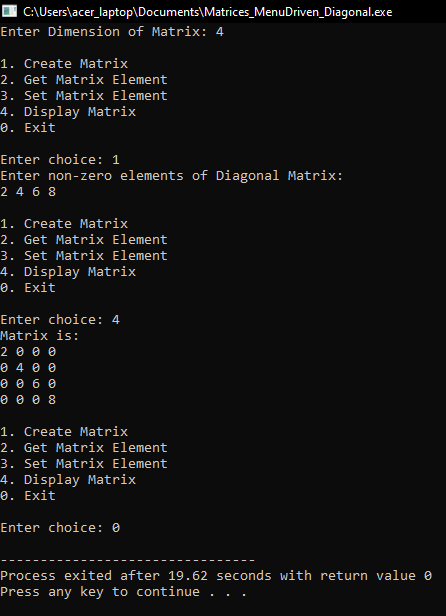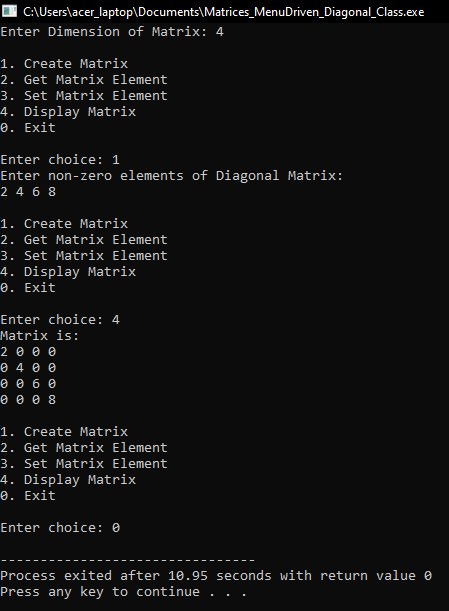# Menu Driven Program for Matrices

## Menu Driven Program for Matrices in C and C++ Language:

In this article, I am going to discuss Menu Driven Program for Matrices in C and C++ Language with Examples. Please read our previous article, where we discussed Toeplitz Matrix in C Language with Examples.

##### Menu Driven Program for Matrices:

We will show for the only diagonal matrix but you can modify the program to create a menu program for other matrices. In our program, there will be 5 choices:

• Create Matrix
• Get Element
• Set Element
• Display Matrix
• Exit

Now, let see the code:

##### Menu Driven Program for Matrices Code in C Language:
```#include <stdio.h>
#include <stdlib.h>

int main ()
{
int n, ch, y, i, j;

printf ("Enter Dimension of Matrix: ");
scanf ("%d", &n);
int *B = (int *) malloc (n * sizeof (int));

do
{
printf ("\n1. Create Matrix\n");
printf ("2. Get Matrix Element\n");
printf ("3. Set Matrix Element\n");
printf ("4. Display Matrix\n");
printf ("0. Exit\n\n");

printf ("Enter choice: ");
scanf ("%d", &ch);

switch (ch)
{
case 1:
printf ("Enter non-zero elements of Diagonal Matrix:\n");
for (int k = 0; k < n; k++)
{
scanf ("%d", &B[k]);
}
break;

case 2:
printf ("Enter Indices: \n");
scanf ("%d%d", &i, &j);
if (i == j)
printf ("%d ", B[i - 1]);
else
printf ("0 ");
break;

case 3:
printf ("Enter row, column and element:\n");
scanf ("%d%d%d", &i, &j, &y);
if (i == j)
B[i - 1] = y;
break;

case 4:
printf ("Matrix is: \n");
for (int r = 0; r < n; r++)
{
for (int c = 0; c < n; c++)
{
if (r == c)
{
printf ("%d ", B[r]);
}
else
{
printf ("0 ");
}
}
printf ("\n");
}
break;

printf ("Enter choice: ");
scanf ("%d", &ch);
}
}
while (ch > 0);

getchar ();
}
```
###### Output:##### Menu Driven Program for Matrices Using Functions:

In our program, there will be 5 choices:

• Create Matrix
• Get Element
• Set Element
• Display Matrix
• Exit

Now, let see the code:

##### Menu Driven Program for Matrices Using Functions Code in C Language:
```#include <stdio.h>
#include <stdlib.h>

int* CreateMatrix (int *B, int n)
{
printf ("Enter non-zero elements of Diagonal Matrix:\n");
for (int k = 0; k < n; k++)
{
scanf ("%d", &B[k]);
}
}

void SetElement (int *B, int y, int i, int j)
{
if (i == j)
B[i - 1] = y;
}

void GetElement (int *B, int i, int j)
{
if (i == j)
printf ("%d ", B[i - 1]);
else
printf ("0 ");
}

void DisplayMatrix (int *B, int n)
{
printf ("Matrix is: \n");
for (int r = 0; r < n; r++)
{
for (int c = 0; c < n; c++)
{
if (r == c)
{
printf ("%d ", B[r]);
}
else
{
printf ("0 ");
}
}
printf ("\n");
}
}

int main ()
{
int n, ch, y, i, j;

printf ("Enter Dimension of Matrix: ");
scanf ("%d", &n);
int *B = (int *) malloc (n * sizeof (int));

do
{
printf ("\n1. Create Matrix\n");
printf ("2. Get Matrix Element\n");
printf ("3. Set Matrix Element\n");
printf ("4. Display Matrix\n");
printf ("0. Exit\n\n");

printf ("Enter choice: ");
scanf ("%d", &ch);

switch (ch)
{
case 1:
CreateMatrix (B, n);
break;

case 2:
printf ("Enter Indices: \n");
scanf ("%d%d", &i, &j);
GetElement (B, i, j);
break;

case 3:
printf ("Enter row, column and element:\n");
scanf ("%d%d%d", &i, &j, &y);
SetElement (B, y, i, j);
break;

case 4:
DisplayMatrix (B, n);
break;

printf ("Enter choice: ");
scanf ("%d", &ch);
}
}
while (ch > 0);
getchar ();
}
```
###### Output:##### Menu Driven Program for Matrices Using Class in C++:

In our program, there will be 5 choices:

• Create Matrix
• Get Element
• Set Element
• Display Matrix
• Exit

Now, let see the code:

##### Menu Driven Program for Matrices Code in C++ Language:
```#include <iostream>
#include <conio.h>
using namespace std;

class Diagonal
{
private:
int *B, n;
public:
Diagonal (int n);
void Create ();
void Get (int i, int j);
void Set (int i, int j, int y);
void Display ();
~Diagonal ();
};

Diagonal::Diagonal (int n)
{
this->n = n;
B = new int[this->n];
}

void Diagonal::Create ()
{
cout << "Enter non-zero elements of Diagonal Matrix:\n";
for (int k = 0; k < n; k++)
{
cin >> B[k];
}
}

void Diagonal::Get (int i, int j)
{
if (i == j)
cout << "%d " << B[i - 1];
else
cout << "0 ";
}

void Diagonal::Set (int i, int j, int y)
{
if (i == j)
B[i - 1] = y;
}

void Diagonal::Display ()
{
cout << "Matrix is: \n";
for (int r = 0; r < n; r++)
{
for (int c = 0; c < n; c++)
{
if (r == c)
{
cout << B[r] << " ";
}
else
{
cout << "0 ";
}
}
cout << "\n";
}
}

Diagonal::~Diagonal ()
{
delete[]B;
}

int main ()
{
int n, ch, y, i, j;

cout << "Enter Dimension of Matrix: ";
cin >> n;
Diagonal dm (n);

do
{
cout << "\n1. Create Matrix\n";
cout << "2. Get Matrix Element\n";
cout << "3. Set Matrix Element\n";
cout << "4. Display Matrix\n";
cout << "0. Exit\n\n";

cout << "Enter choice: ";
cin >> ch;

switch (ch)
{
case 1:
dm.Create ();
break;

case 2:
cout << "Enter Indices: \n";
cin >> i >> j;
dm.Get (i, j);
break;

case 3:
cout << "Enter row, column and element:\n";
cin >> i >> j >> y;
dm.Set (i, j, y);
break;

case 4:
dm.Display ();
break;

cout << "Enter choice: ";
cin >> ch;
}
}
while (ch > 0);
getchar ();
}
```
###### Output:Here, in this article, I try to explain Menu Driven Program for Matrices in C and C++ Language with Examples and I hope you enjoy this Menu Driven Program for Matrices in C and C++ Language with Examples article.